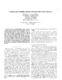## Capacity and Variability Analysis of the IEEE 802.11 MAC Protocol##### Files
CS-TR-4475.ps(2.85 MB)
{\em Packet error} in the IEEE 802.11 network %which is due to non-ideal channel condition and wireless device variability, is one source of performance degradation and its variability. %Therefore the effect of packet errors, along with {\em collision %avoidance} and {\em hidden terminals}, is among the most important %considerations in performance anaylsis of the 802.11 MAC protocol. Most of the previous works study how {\em collision avoidance} and {\em hidden terminals} affect 802.11 performance metrics, such as probability of a collision and saturation throughput. In this paper we focus on the effect of packet errors on capacity and variability of the 802.11 MAC protocol. We develope a new analytical model, called $p_e$-Model, by extending the existing model (Tay and Chua's model) to incorporate {\em packet error probability} $p_e$. With $p_e$-Model, we successfully analyze capacity and variability of the 802.11 MAC protocol. The variability analysis shows that increasing packet error probability by $\Delta p_e$ has more effect on saturation throughput, than adding $0.5 W \Delta p_e$ stations, where $W$ is the minimum contention window size, We also show the numerical validation of $p_e$-Model with 802.11 MAC-level simulator. (UMIACS-TR-2003-45)